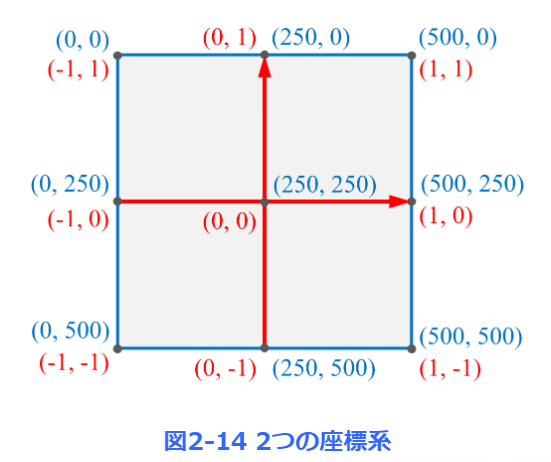# [JAVA] Introduction to SWING

### Method type

drawLine(x1, y1, x2, y2) Draw a straight line from the point (x1, y1) to the point (x2, y2).

#### drawString ("string", x, y)

Place the character string at the position where the point (x, y) is at the lower left.

drawRect(x, y, w, h) Draw a rectangle with a width w and a height h, with the point (x, y) at the upper left.

fillRect(x, y, w, h) With the point (x, y) at the upper left, draw a rectangle with a width w and a height h, and fill the inside.

drawOval(x, y, w, h) Draw an ellipse inscribed in a rectangle with a width w and a height h, with the point (x, y) at the upper left.

fillOval(x, y, w, h) With the point (x, y) at the upper left, draw an ellipse inscribed in a rectangle with a width w and a height h, and fill the inside.

drawPolygon(x, y, n) An array of x coordinates x and an array of y coordinates Draw a polygon consisting of n points defined by y.

fillPolygon(x, y, n) An array of x coordinates x and an array of y coordinates Draw a polygon consisting of n points defined by y and fill the inside.

drawPolyline(x, y, n) An array of x coordinates x and an array of y coordinates y Draw the n points defined by y as a continuous straight line.

drawRoundRect(x,y,w,h,aw,ah) Draw a contour rectangle with rounded corners.

fillRoundRect(x,y,w,h,aw,ah) Draw a contour rectangle with rounded corners and fill the interior.

### Class type

JFrame Class to display a frame (window) on the screen of the terminal

JPanel Class to place GUI parts such as figures in the frame

Container Container class for holding GUI parts

Graphics Class to draw in the frame

Color Color handling class

### Coordinates on the window